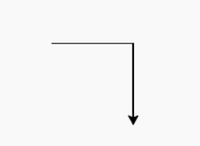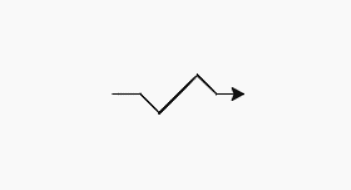# turtle.pos() method in Python

The Turtle module provides turtle graphics primitives, in both object-oriented and procedure-oriented ways. Because it uses Tkinter for the underlying graphics, it needs a version of Python installed with Tk support.

## turtle.pos()

This method is used to find the turtle’s current location (x, y), as a Vec2D-vector. This method has the Aliases: pos | position.

Syntax: turtle.pos() or  turtle.position()

Return: turtle’s current location in terms of (x, y) coordinate

This function does not require any argument and returns the current position of the turtle in the format (x,y) where x and y represent the 2D vector. The default value is (0.0, 0.0).

Below is the implementation of the above method with some examples :

Example 1 :

## Python3

 `# import turtle package ` `import` `turtle  ` ` `  `# print the default  ` `# position i.e; (0.0, 0.0) ` `print``(turtle.pos()) ` ` `  `# forward turtle by 150 pixels ` `turtle.forward(``150``) ` ` `  `# print current position  ` `# i.e; (150.0, 0.0) ` `print``(turtle.pos()) ` ` `  `# forward turtle by 150 pixels ` `# after taking turn right ` `# by 90 degrees ` `turtle.right(``90``) ` `turtle.forward(``150``) ` ` `  `# print position (after next move) ` `# i.e; (150.0, -150.0) ` `print``(turtle.pos())`

Output:```(0.0, 0.0)
(150.0, 0.0)
(150.0, -150.0)
```

Example 2:

## Python3

 `# import turtle package ` `import` `turtle  ` ` `  `# print position (by default) ` `# i.e; (0.0, 0.0) ` `print``(turtle.pos()) ` ` `  `# turtle move forward  ` `# by 40 pixels ` `turtle.forward(``40``) ` ` `  `# print position (after move) ` `# i.e; (150.0, 0.0) ` `print``(turtle.position()) ` ` `  `# turtle move forward by 40 pixels ` `# after taking right turn  ` `# by 45 degrees ` `turtle.right(``45``) ` `turtle.forward(``40``) ` ` `  `# print position ` `# (after next move)  ` `print``(turtle.pos()) ` ` `  `# turtle move forward by 80  ` `# pixels after taking left ` `# turn by 90 degrees ` `turtle.left(``90``) ` `turtle.forward(``80``) ` ` `  `# print position ` `# (after next move)  ` `print``(turtle.pos()) ` ` `  `# turtle move forward  ` `# by 40 pixels after taking  ` `# right turn by 90 degrees ` `turtle.right(``90``) ` `turtle.forward(``40``) ` ` `  `# print position (after next move)  ` `print``(turtle.position()) ` ` `  `# turtle move forward by  ` `# 40 pixels after taking  ` `# left turn by 45 degrees ` `turtle.left(``45``) ` `turtle.forward(``40``) ` ` `  `# print position  ` `# (after final move)  ` `print``(turtle.pos())`

Output :```(0.0, 0.0)
(40.0, 0.0)
(68.2842712475, -28.2842712475)
(124.852813742, 28.2842712475)
(153.13708499, 0.0)
(193.13708499, 0.0)
```

My Personal Notes arrow_drop_upCheck out this Author's contributed articles.

If you like GeeksforGeeks and would like to contribute, you can also write an article using contribute.geeksforgeeks.org or mail your article to contribute@geeksforgeeks.org. See your article appearing on the GeeksforGeeks main page and help other Geeks.

Please Improve this article if you find anything incorrect by clicking on the "Improve Article" button below.

Article Tags :

Be the First to upvote.

Please write to us at contribute@geeksforgeeks.org to report any issue with the above content.